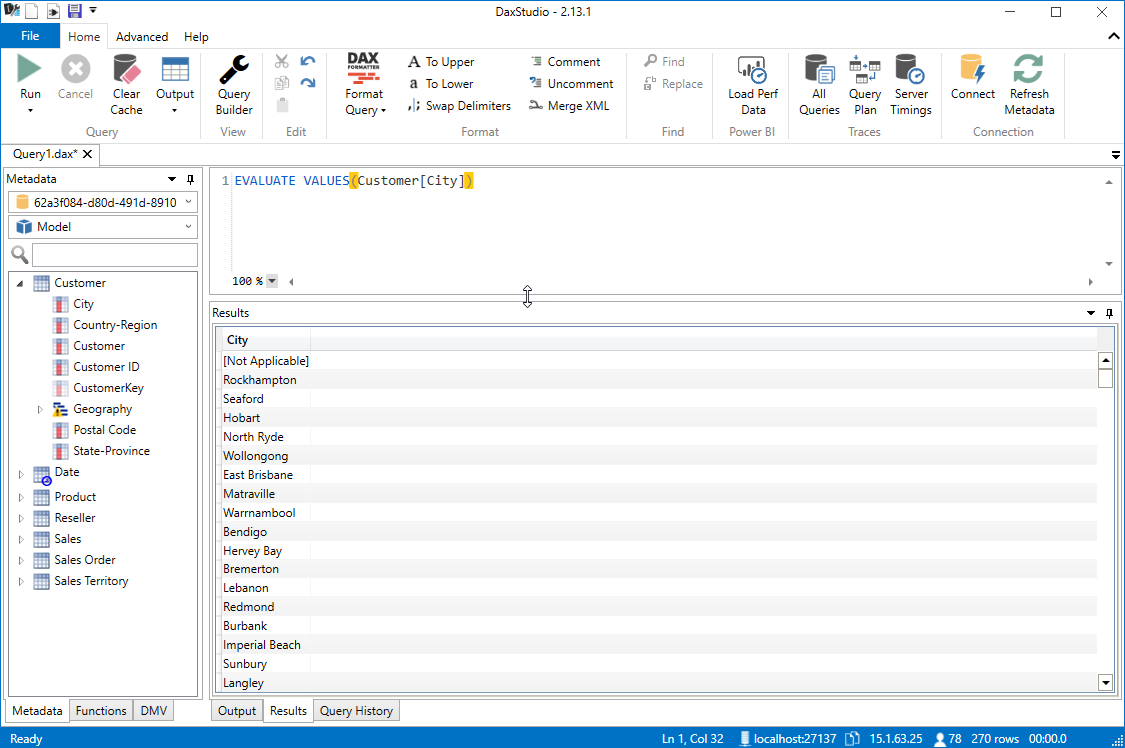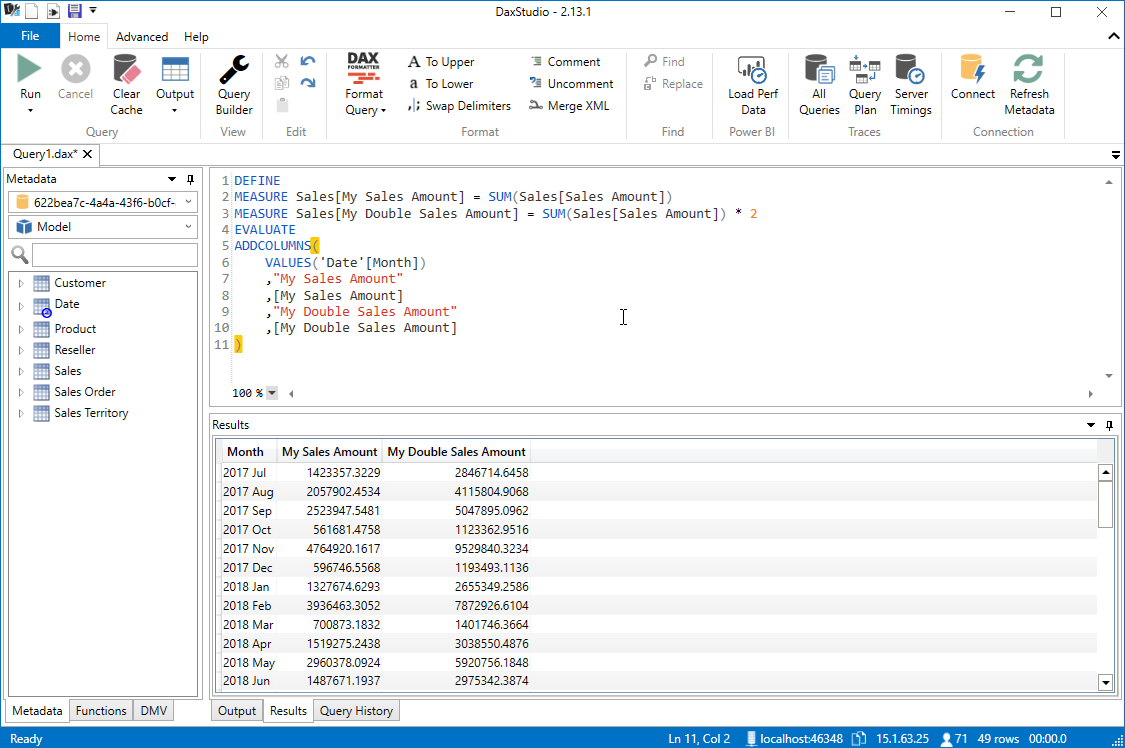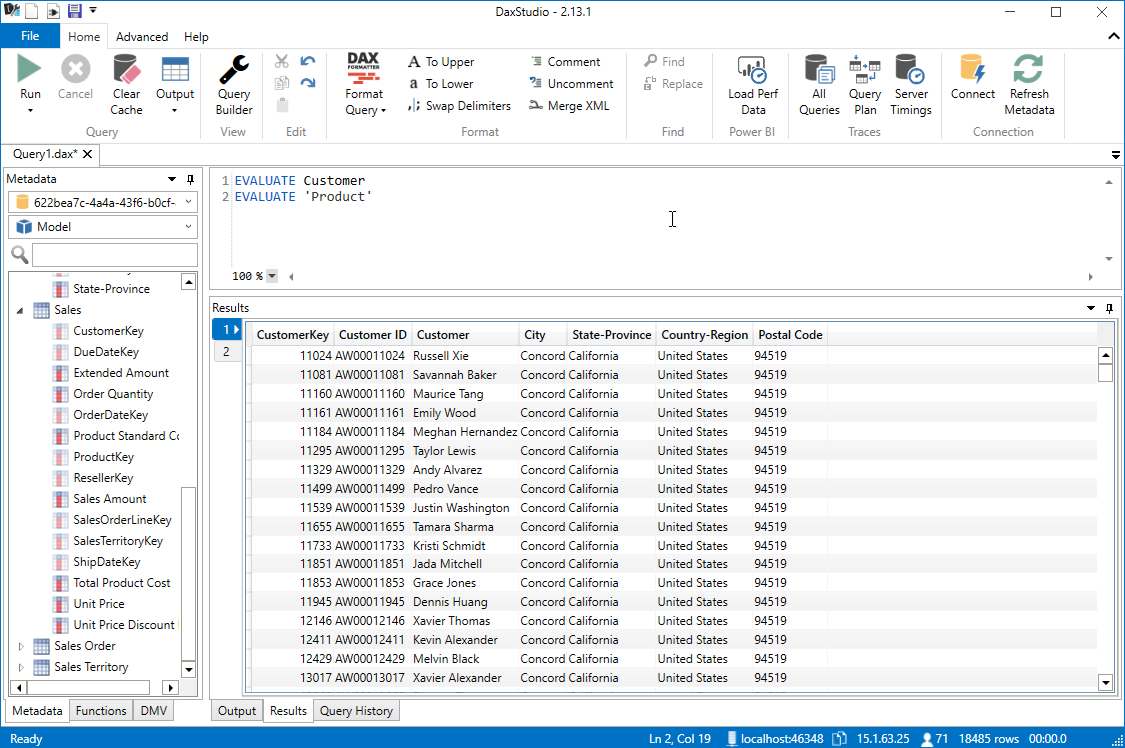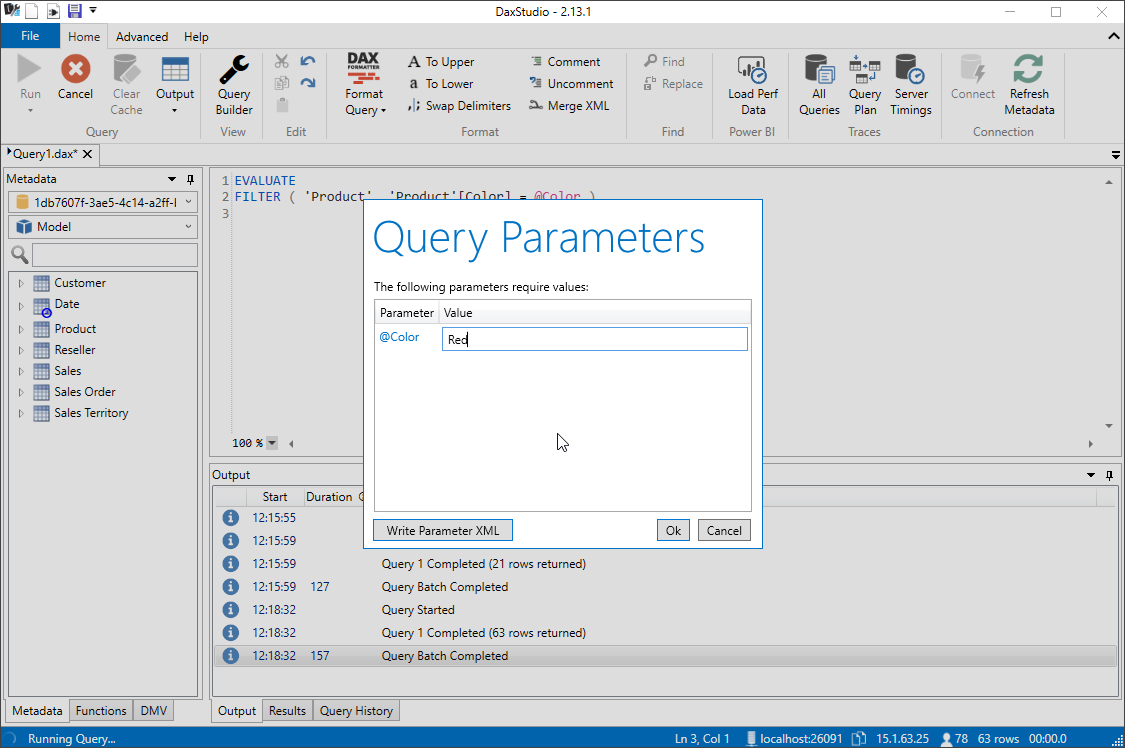# Writing DAX Queries

DAX Queries have quite a simple structure. Microsoft describes the query syntax in their documentation here. But in this guide we are going to take a very practical, example based approach.

If you want to follow along and try out these queries yourself all you need is:

The simplest way to get started after installing both DAX Studio and Power BI Desktop is to open the Adventure Works 2020.pbix file, then click on External Tools and launch DAX Studio from there.

tip

For more details on how you can connect to your particular data model check out the tutorial on Getting Connected

## Basic Queries​

The simplest form of DAX query is `EVALUATE <table expression>`

So you can run `EVALUATE Customer` to output all the rows in the customer tableBut you don't just have to use a table name, you can use any function that returns a table. To get a distinct list of all the values in a column you can use the VALUES function which returns a table with a single row with all the unique values from the specified column.

``EVALUATEVALUES ( Customer[City] )``Or if you don't want every row in the table you could use the CALCULATETABLE function to only return rows that meet a given criteria.

``EVALUATECALCULATETABLE ( Customer, Customer[City] = "Redmond" )``

You can even combine the VALUES and CALCULATETABLE functions to get a list of all Cities that have a first character of "R"

``EVALUATECALCULATETABLE ( VALUES ( Customer[City] ), LEFT ( Customer[City], 1 ) = "R" )``

## Sorting Results​

If we continue on with the previous example you will see that the results come back in a random order. If we want our query to sort the results we can add an optional `ORDER BY` clause to the end of the query. So if we wanted to sort the results by the city name we would do the following:

``EVALUATECALCULATETABLE ( VALUES ( Customer[City] ), LEFT ( Customer[City], 1 ) = "R" )ORDER BY Customer[City]``

NOTE: Some client tools (like Power BI Desktop) will generate an `ORDER BY` clause for you based on the properties set in your data model

To add a calculation to your query like measures and variables you would use the optional `DEFINE` keyword at the start of your query

To define a new measure in your query which sums the value of the existing `Sales[Sales Amount]` column you would write the following:

``DEFINE    MEASURE Sales[My Sales Amount] =        SUM ( Sales[Sales Amount] )EVALUATEADDCOLUMNS ( VALUES ( 'Date'[Month] ), "My Sales Amount", [My Sales Amount] )``

To define multiple measures you can add multiple blocks of `MEASURE <table>[<measure name>] = <expression>`

``DEFINE    MEASURE Sales[My Sales Amount] =        SUM ( Sales[Sales Amount] )    MEASURE Sales[My Double Sales Amount] =        SUM ( Sales[Sales Amount] ) * 2EVALUATEADDCOLUMNS (    VALUES ( 'Date'[Month] ),    "My Sales Amount", [My Sales Amount],    "My Double Sales Amount", [My Double Sales Amount])``## Returning a single value​

Sometimes you may just want to return the result of a measure. But measures return a single scalar value not a table, so if you try to write the following it will produce a syntax error

``EVALUATESUM ( Sales[Sales Amount] )``

We can fix this by using the table constructor syntax and wrapping the measure in curly braces `{ }`

``EVALUATE{ SUM ( Sales[Sales Amount] ) }``

For older versions of the tabular engine which do not support the table constructor syntax we can use the ROW function

``EVALUATEROW ( "Sales Amount", [Sales Amount] )``

You can also mix this with the `DEFINE` clause to create a measure expression and then return a single value

``DEFINE    MEASURE Sales[Total Sales] =        SUM ( Sales[Sales Amount] )EVALUATE{ [Total Sales] }``

## Selecting columns from multiple tables​

The easiest way to generate a query using columns from multiple tables is to use the SUMMARIZECOLUMNS function. This function takes a list of columns, followed by an optional list of table expressions to use as filters, followed by an optional list of measures/expressions.

NOTE: It is strongly recommended to always use a measure or expression of some sort with the `SUMMARIZECOLUMNS` function if you don't do this the function will generate a large crossjoin of all possible combinations of every value in the specified columns which is not normally useful

``EVALUATESUMMARIZECOLUMNS (    Product[Color],    Reseller[Business Type],    FILTER ( ALL ( 'Product'[List Price] ), 'Product'[List Price] > 150.00 ),    TREATAS ( { "Accessories", "Bikes" }, 'Product'[Category] ),    "Total Sales", SUM ( Sales[Sales Amount] ))``

## Multiple Resultsets​

DAX queries also allow for the return of multiple recordsets within a given batch

So you can execute the following:

``EVALUATECustomerEVALUATE'Product'``And you will get 2 tabs returned in DAX Studio, one with the contents of the Customer table and the other with the contents of the Product table.

But note that within a single batch, although you can have multiple `EVALUATE` statements you can only have a single `DEFINE` statement. So you would need to declare all your calculations in that one block.

``DEFINE    MEASURE Sales[Total Sales] =        SUM ( Sales[Sales Amount] )    MEASURE Sales[Total Cost] =        SUMX ( Sales, Sales[Unit Price] * Sales[Order Quantity] )EVALUATE{ [Total Sales] }EVALUATE{ [Total Cost] }``## Using Parameters in Queries​

One of the unique features that DAX Studio has is the support for parameterized queries

To add a parameter to a DAX query you can start with a query that includes a filter such as the following:

``EVALUATEFILTER ( 'Product', 'Product'[Color] = "Red" )``

And then replace the reference to `"Red"` with a parameter called `@Color`

``EVALUATEFILTER ( 'Product', 'Product'[Color] = @Color )``

When you execute a query with a parameter, DAX Studio will prompt you for the parameter to use